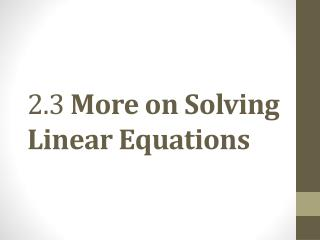DownloadDownload Presentation2.3 More on Solving Linear Equations

# 2.3 More on Solving Linear Equations

Télécharger la présentation## 2.3 More on Solving Linear Equations

- - - - - - - - - - - - - - - - - - - - - - - - - - - E N D - - - - - - - - - - - - - - - - - - - - - - - - - - -
##### Presentation Transcript

1. 2.3 More on Solving Linear Equations

2. Objective 1 Learn and use the four steps for solving a linear equation. Slide 2.3-3

3. Learn and use the four steps for solving a linear equation. We solve more complicated equations using the following four-step method. Solving a Linear Equation Step 1:Simplify each side separately.Clear parentheses, fractions, and decimals, using the distributive property as needed, and combine all like terms. Step 2:Isolate the variable term on one side.Use the addition property if necessary so that the variable term is on one side of the equation and a number is on the other. Step 3:Isolate the variable.Use the multiplication property if necessary to get the equation in the form x = a number. (Other letters may be used for variables.) Step 4:Check.Substitute the proposed solution into the originalequation to see if a true statement results. Slide 2.3-4

4. CLASSROOM EXAMPLE 1 Applying Both Properties of Equality to Solve an Equation Solve. Solution: Check: The solution set of the equation is {−3}. Slide 2.3-5

5. CLASSROOM EXAMPLE 2 Applying Both Properties of Equality to Solve an Equation Solve. Check: Solution: The solution set of the equation is {1}. Remember that the variable can be isolated on either side of the equation. There are often several equally correct ways to solve an equation. Slide 2.3-6

6. Using Four Steps to Solve an Equation CLASSROOM EXAMPLE 3 Solve. Solution: Check: The solution set of the equation is {−1}. Slide 2.3-7

7. The solution set of the equation is Using the Four Steps to Solve an Equation CLASSROOM EXAMPLE 4 Solve. Solution: Check: Slide 2.3-8

8. Learn and use the four steps for solving a linear equation. (cont’d) Be very careful with signs when solving an equation like the one in the previous example. When clearing parentheses in the expression remember that the − sign acts like a factor of −1 and affects the sign of every term within the parentheses. Slide 2.3-9

9. Using the Four Steps to Solve an Equation CLASSROOM EXAMPLE 5 Solve. Solution: Check: The solution set of the equation is {0}. Slide 2.3-10

10. Objective 2 Solve equations with fractions or decimals as coefficients. Slide 2.3-11

11. Solve equations with fractions or decimals as coefficients. We clear an equation of fractions by multiplying each side by the least common denominator (LCD) of all the fractions in the equation. It is a good idea to do this to avoid messy computations. When clearing an equation of fractions, be sure to multiply every term on each side of the equation by the LCD. Slide 2.3-12

12. Solving an Equation with Fractions as Coefficients CLASSROOM EXAMPLE 6 Solve. Solution: Check: The solution set of the equation is {−7}. Slide 2.3-13

13. Solving an Equation with Fractions as Coefficients CLASSROOM EXAMPLE 7 Solve. Solution: The solution set of the equation is {5}. Slide 2.3-14

14. CLASSROOM EXAMPLE 7 Solving an Equation with Fractions as Coefficients (cont’d) Check: The solution set of the equation is {5}. Slide 2.3-15

15. Solving an Equation with Decimals as Coefficients CLASSROOM EXAMPLE 8 Solve. Solution: Multiplying by 10 is the same as moving the decimal point one place to the right. Likewise, multiplying by 100 is the same as moving the decimal point two places to the right. Slide 2.3-16

16. CLASSROOM EXAMPLE 8 Solving an Equation with Decimals as Coefficients (cont’d) Check: The solution set of the equation is {−2}. Slide 2.3-17

17. Objective 3 Solve equations with no solution or infinitely many solutions. Slide 2.3-18

18. Solve equations with no solution or infinitely many solutions. Each equation that we have solved so far has had exactly one solution. An equation with exactly one solution is a conditional equation because it is only true under certain conditions. Sometimes equations may have no solution or infinitely many solutions. Slide 2.3-19

19. DO NOT write { 0 } as the solution set. There are infinitely many other solutions. For { 0 } to be the solution set, the last line must include a variable, such as x, and read x = 0, not 0 = 0. CLASSROOM EXAMPLE 9 Solving an Equation That Has Infinitely Many Solutions Solve. Solution: The variable has “disappeared.” Since the last statement is true, any real number is a solution. An equation with both sides exactly the same, is called an identity. An identity is true for all replacements of the variables. We write the solution set as {all real numbers}. Slide 2.3-20

20. Solving an Equation That Has No Solution CLASSROOM EXAMPLE 10 Solve. Solution: Again, the variable has “disappeared,” but this time a false statement results. When this happens in solving an equation, it indicates that the equation has no solution and is called a contradiction. Its solution set is the empty set, or null set, symbolizedØ. DO NOT write { Ø } to represent the empty set. Slide 2.3-21

21. Solve equations with no solution or infinitely many solutions. The following table summarizes the solution sets of the three types of equations presented so far. Slide 2.3-22

22. Objective 4 Write expressions for two related unknown quantities. Slide 2.3-23

23. Translating a Phrase into an Algebraic Expression CLASSROOM EXAMPLE 11 Two numbers have a product of 36. If one of the numbers is represented by x, find an expression for the other number. Solution: Slide 2.3-24